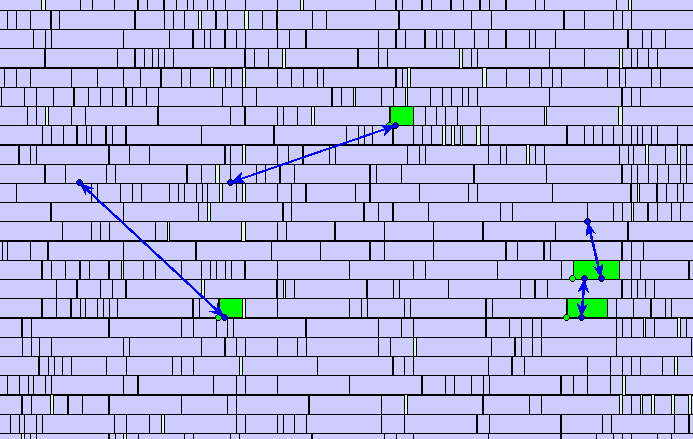﻿ NanDigits: Netlist Processing Platform GOF ECO GOF Formal

## Clock Gating Cell or MUX

Clock gating is a technique used in logic design to save area and reduce power consumption when the number of flops grouped together exceeds a certain threshold. This threshold is calculated by comparing the area ratio of a MUX to that of a clock gating cell. If the area of the clock gating cell is less than that of a MUX multiplied by the number of flops, then it is more area-efficient to use clock gating logic. If the area of a clock gating cell is less than the area of a MUX multiplied by the number of flops, then it is more area-efficient to use clock gating logic. This clock gating logic can be represented as a MUX feedback format, as shown in Figure 1.Figure 1: Clock Gating vs MUX

Gate array spare cell ECO shows that when using more than three flops with one clock gating, it is more area-efficient to use clock gating logic instead of MUX logic. The table of tile numbers used in gate array cells indicates that the left clock gating logic in Figure 1 occupies 32 tiles (5X4+12), whereas the right MUX logic uses 40 tiles (5X4+5X4). Therefore, grouping four flops using a clock gating cell can save eight tiles.

1GFILL1GTIE GINVD1 GND2D1 GNR2D1
2GFILL2GBUFD1 GAN2D1 GOR2D1 GAOI21D1 GDN3D1
3GFILL3GAO21D1 GAN4D1 GOR4D1
4GFILL4GINVD8 GAN2D4
5GFILL5GMUX2D1 GXOR2D1 GXNOR2D1
6GFILL6GBUFD8 GSDFFRQD1 GSDFFSQD1
8GFILL8GINVD16
12GFILL12GCKLNQD6

Table: Gate Array Tiles Number for Cells

The situation becomes more complex when the gates are mapped to actual gate array spare fillers. This is because the clock gating cell, which uses 12 tiles, has much less distribution than the 5-tile MUX. As shown in Figure 2, the 12-tile filler that is found is relatively far from the flops and can impact the timing closure.Figure 2: Placement for One Clock Gating Cell Drives Four Flops

On the other hand, MUX logic has more resources to find close-by 5-tile MUXs, making the placement more concentrated and the connections shorter. Thus, in this case, it is more practical to select MUX logic over clock gating logic.Figure 3: Placement for Four MUXs Drive Four Flops

GOF provides an API called "convert_gated_clocks" which can be used to convert clock gating logic to MUX feedback format logic. For more details on how to use this API, please refer to the User Manual.

Follow us:NanDigits.com US | NanDigits.cn China